# HBSE 6th Class Maths Solutions Chapter 7 Fractions Ex 7.1

Haryana State Board HBSE 6th Class Maths Solutions Chapter 7 Fractions Ex 7.1 Textbook Exercise Questions and Answers.

## Haryana Board 6th Class Maths Solutions Chapter 7 Fractions Exercise 7.1

Question 1.
Give the fraction representing the shaded partion?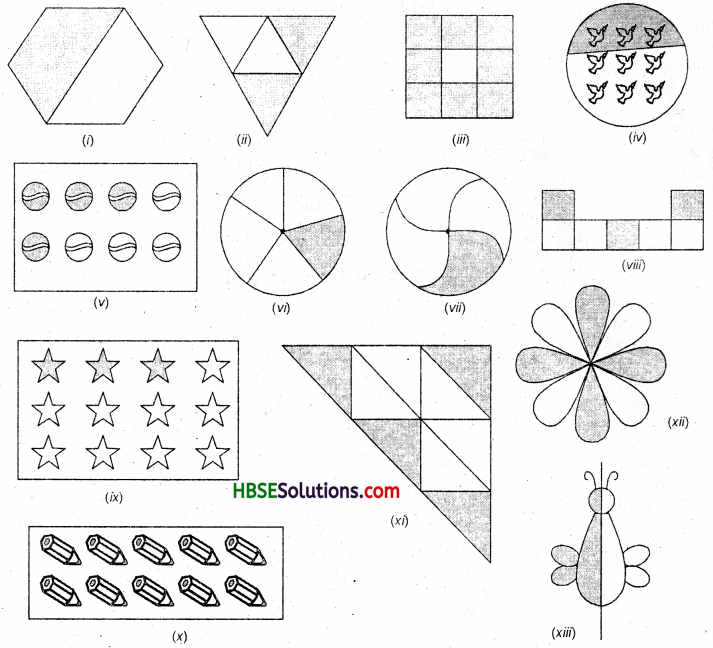Solution: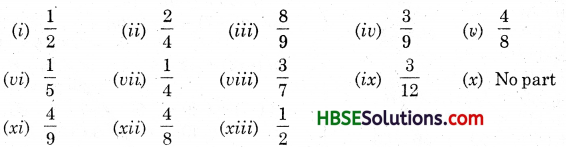Question 2.
Colour the part according to the fraction given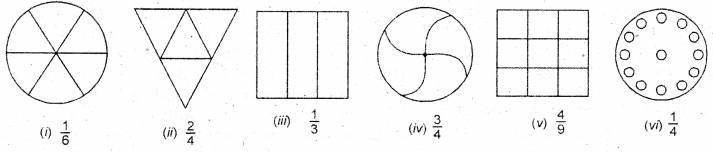Solution: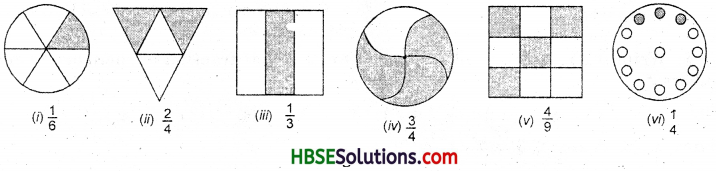Question 3.
Identify the error, if any ?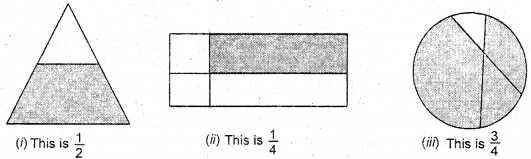Solution:
(i) This is not $$\frac{1}{2}$$, because the two parts are not equal.
(ii) This is not $$\frac{1}{4}$$ , because the four parts are not equal.
(iii) This is not $$\frac{3}{4}$$, because the four parts are not equal.Question 4.
What fraction of a day is 8 hours ?
Solution:
∵ A day has 24 hours
∴ 8 hours = $$\frac{8}{24}=\frac{1}{3}$$

Question 5.
What fraction of an hour is 40 minutes ?
Solution:
∵ An hour has 60 minutes
∴ 40 minutes = $$\frac{40}{60}=\frac{2}{3}$$

Question 6.
Arya, Abhimanyu and Vivek share for lunch. Arya brought two sandwiches, one made of vegetable and one of jam. The other two boys forget theirs lunch. Arya agree to share his sandwiches so that each person will have an equal shares of each sandwich.
(a) How can Arya divide his sandwiches so that each person has an equal share ?
(b) What part of a sandwich will each boy receive ?
Solution:
(a) Arya can divide each sandwich into three equal parts, total 6 parts.
(b) Each boy will receive l/3rd part of each sandwich i.e. each gets 2 parts.

Question 7.
Kanchan has three frocks that she wears while playing. The material is good, but the colours are faded. Her mother buys same blue dye and uses it on two of the frocks. What fraction of Kanchan’s playing frocks did her mother dye ?
Solution:
Her mother dyed 2/3rd of Kanchan’s playing frocks.

Question 8.
Write the natural numbers from numbers ?
Solution: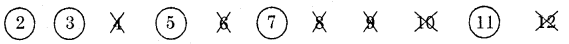Prime numbers are : 2, 3, 5, 7, 11 i.e., 5.
Hence, $$\frac{5}{11}$$ fraction are prime numbers.

Question 9.
Write the natural numbers from 102 to 103 numbers ? What fraction of them are prime numbers?
Solution: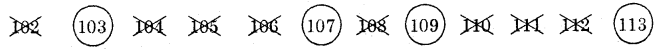Prime numbers are : 103, 107, 109, 113 i.e., 4
Total natural numbers are 12.
Hence, $$\frac{4}{12}=\frac{1}{3}$$ fraction are prime numbers.Question 10.
What fraction of these circles have X’s in them ?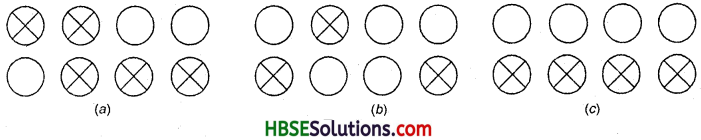Solution:
(a) $$\frac{5}{8}$$
(b) $$\frac{3}{8}$$
(c) $$\frac{4}{8}$$

Question 11.
Dinesh, Sumit, Ram, Joy, Marshal, Imran, Jayant, Babu, Kabir and Rohan decide to play basketball. The first five boys are of one team and the rest are of the other team.
(a) What fraction of the boys is of the first team ?
(b) What, does this fraction mean ? Are equal parts involved ? If so, equal in what sense?
Solution:
(a) ∵ There are ten boys in all. and no. of boys in the first team = 5
∴ Fraction of the boys in the first team
= $$\frac{5}{10}=\frac{1}{2}$$

(b) Fraction $$\frac{1}{2}$$ means that half the boys are in the first team. Yes, equal parts are involved.
This shows that five boys are in the first team and five boys are in the second team.Question 12.
Kristin receive a C.D. Player for her birthday. She has been collecting C.D’s since then. She bought 3 C.D’s and received 5 others as gifts. What fraction of her total C.D’s did she buy ?
Solution:
Total number of C.D’s she has = 3 + 5 = 8
Number of C.D’s bought by her = 3
Hence, the required fraction of C.D’s she bought
= $$\frac{3}{8}$$